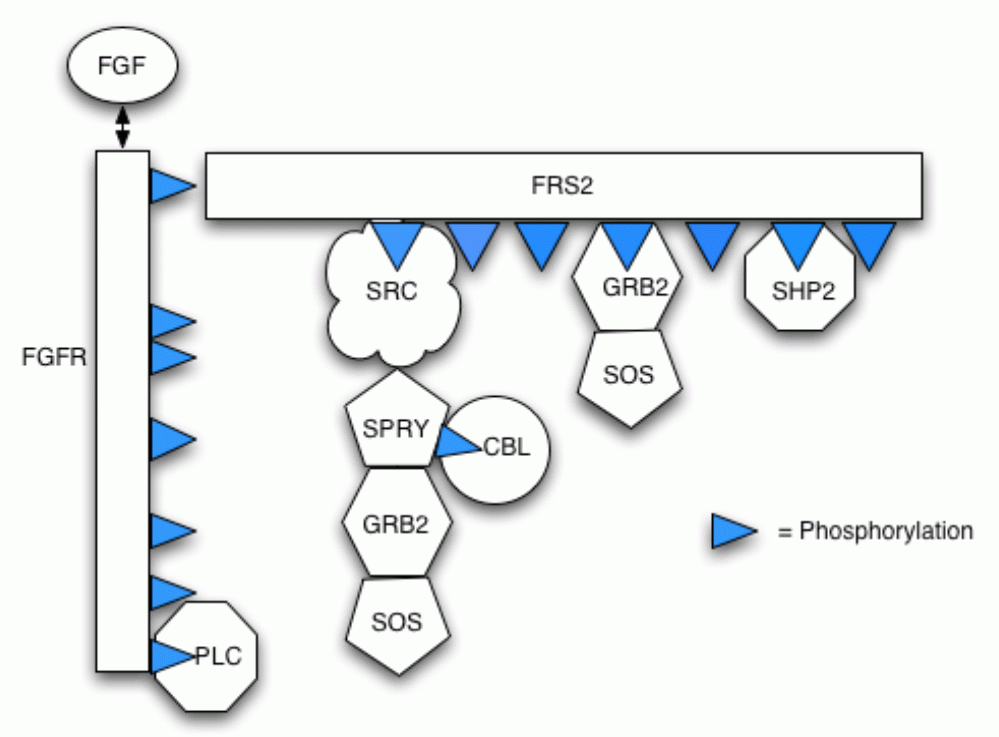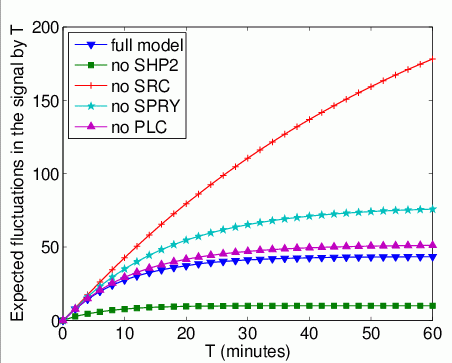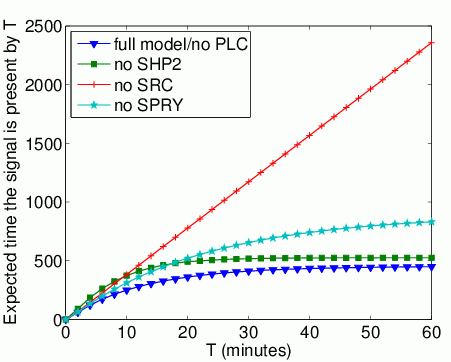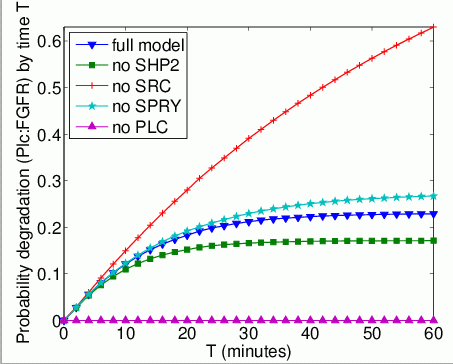# FGF (Fibroblast Growth Factor) Signalling

### Contents

Related publications: [HKN+06, HKN+08]

### Introduction

Fibroblast Growth Factors (FGF) are a family of proteins which play a key role in the process of cell signalling in a variety of contexts, for example wound healing. The mechanisms of the FGF signalling pathway are complex and not yet fully understood. In the case study we analyse a model of the pathway based on literature-derived information regarding the early stages of FGF signal propagation and which incorporates several features that have been reported to negatively regulate this propagation.

### The Pathway

Our model incorporates protein-protein interactions (including competition for partners), phosphorylation and dephosphorylation, protein complex relocation and protein complex degradation (via ubiquitin-mediated proteolysis). The follow figure illustrates the different components in the pathway and their possible bindings.Below is a list of the reactions included in the model.

• 1. An FGF ligand binds to an FGF receptor (FGFR) creating a complex of FGF and FGFR.
• 2. The existence of this FGF:FGFR dimer leads to phosphorylation of FGFR on two residues Y653 and Y654 in the activation loop of the receptor.
• 3. The dual Y653/654 form of the receptor leads to phosphorylation of other FGFR receptor residues: Y663, Y583, Y585, Y766 (in this model we only consider Y766 further).
• 4. and 5. The dual Y653/654 form of the receptor also leads to phosphorylation of the FGFR substrate FRS2, which binds to both the phosphorylated and dephosphorylated forms of the FGFR.
• 6. FRS2 can also be dephosphorylated by a phosphotase, denoted Shp2.
• 7. A number of effector proteins interact with the phosphorylated form of FRS2. In this model we include Src, Grb2:Sos and Shp2.
• 8. and 9. In these steps we incorporate two methods of attenuating signal propagation by removal (i.e. relocation) of components. In step 8. we assume that Src associated with the phosphorylated FRS2 leads to relocation (i.e. endocytosis and/or degradation of FGFR:FRS2). In step 9. we assume that Plc bound to Y766 of FGFR leads to relocation/degradation of FGFR.
• 10. The signal attenuator Spry is a known inhibitor of FGFR signalling and is synthesised in response to FGFR signalling. Here we include a variable to regulate the concentration of Spry protein in a time dependent manner.
• 11. We incorporate the association of Spry with Src and concomitant phosphorylation of Spry residue Y55.
• 12. The Y55 phosphorylated form of Spry binds Cbl , which leads to ubiquitin modification of FRS2 and a degradation of FRS2 through ubiquitin mediated proteolysis.
• 13. Y55P form of Spry is dephosphorylated by Shp2 bound to FRS2 Y247P.
• 14. Grb2 binds the Y55P form of Spry. In our model Spry competes with FRS2 for Grb2 as has been suggested from some studies in the literature.

The full details of the reactions can be found here.

Note that this model is not intended to and cannot be a fully accurate representation of a real-world FGF signalling pathway. Its primary purpose at this stage of development is as a tool to evaluate biological hypotheses that are not easily obtained by intuition or manual methods. To this end, the model is an abstraction as argued in [RS02].

We explicitly draw attention to the following issues. The reactions selected are based upon their current biological interest rather than complete understanding of the components of FGF signalling. Indeed, at this stage we have ignored many reactions that could prove significant in regulation of FGFR signalling in real cells. However, the design permits the incorporation of further modifications to the core model as biological understanding advances. The model is idealised in that it does not take into account variations in composition, affinities or rate constants that might occur in different cell types or physiological conditions. However, a useful computational modelling approach should accommodate future quantitative or qualitative modifications to the core model, and we explicitly address this issue by evaluation of parameter dependencies below.

The model is based upon literature-defined events. There is always the possibility that the reported biological significance of these processes reflects the experimental context rather than the normal situation. For example, the significance of Plc in the relocation of FGFR signalling complexes has been the subject of some debate, as has the action of Spry. As we demonstrate in this paper, the model as presented provides the ability to ask what if questions which should inform the biological debate.

### The PRISM Model

We now describe the specification in PRISM of the FGF model from the previous section. Each of the basic elements of the pathway, including all the possible compounds and receptors residues (FGF, FGFR, FRS2, Plc, Src, Spry, Sos, Grb2, Cbl and Shp2) is represented by a separate PRISM module and synchronisation between modules is used to model reactions which involve interactions between multiple elements. However, the different forms which each can take (for example, which other compounds it is bound to) are represented by one or more variables within the module. Our model represents a single instance of the signalling pathway, i.e. there can be at most one of each compound.

The study of a single instance of the pathway is also motivated by the fact that the same signal dynamics were obtained for a model where the concentrations were set to 100. Details of this model are available from here - note that this study uses the stochastic pi-calculus and the simulation tool BioSpi.

We constructed several models. In the first model, representing the full system, we suppose that initially FGF, unbound and unphosphorylated FGFR, unphosphorylated FRS2, unbound Src, Grb2, Cbl, Plc and Sos are all present in the system (Spry arrives into the system with the half-time of 10 minutes).

Subsequently, we constructed additional four models in order to investigate the roles of the various components of the activated receptor complex in controlling signalling dynamics. This is done by building modified models of the pathway where certain components are omitted (Shp2, Src, Spry or Plc). This is easily achieved in the PRISM model by just changing the initial value of the component under study.

The PRISM model for the pathway is given below.

```// FGF model
// gxn/dxp 10/02/06

// model is a CTMC
ctmc

// formulae
formula frs = relocFRS2=0 & degFRS2=0; // frs2 not degraded or relocated
formula fgfr = degFGFR=0; // fgfr not degraded or relocated

//-----------------------------------------------------------------------
// modules for the different components

module FGF

FGF : [0..1] init 1; // free

// fgfr+fgf <-> fgfr:fgf 
[fgf_bind] FGF=1 -> (FGF'=0);
[fgf_rel]  FGF=0 -> (FGF'=1);

endmodule

module FGFR

FGFR     : [0..1] init 1; // FGFR unbound to FRS2
degFGFR  : [0..1] init 0; // FGFR degraded
FGFR_FGF : [0..1] init 0; // FGF bound
FGFR_PLC : [0..1] init 0; // PLC bound
// phosporilation of receptors
Y653P : [0..1] init 0;
Y654P : [0..1] init 0;
Y766P : [0..1] init 0;

// fgfr+fgf <-> fgfr:fgf 
[fgf_bind] fgfr & FGFR_FGF=0 -> 5000  : (FGFR_FGF'=1);
[fgf_rel]  fgfr & FGFR_FGF=1 -> 0.002 : (FGFR_FGF'=0);
// phosporilation of receptors
[] fgfr & FGFR_FGF=1 & Y653P=0 -> 0.1 : (Y653P'=1); // FGFR653 -> FGFR653P 
[] fgfr & FGFR_FGF=1 & Y654P=0 -> 0.1 : (Y654P'=1); // FGFR654 -> FGFR654P 
[] fgfr & Y653P=1 & Y654P=1 & Y766P=0 -> 70 : (Y766P'=1); // FGFR766 -> FGFR766P 
// fgfr+frs2 <-> fgfr:frs2 
[fgfr_bind] fgfr & FGFR=1 -> (FGFR'=0);
[fgfr_rel]  fgfr & FGFR=0 -> (FGFR'=1);
// PLC + FGFR766P <-> PLC:FGFR 
[plc_bind] fgfr & Y766P=1 & FGFR_PLC=0 ->   10 : (FGFR_PLC'=1);
[plc_rel]  fgfr & FGFR_PLC=1           -> 0.02 : (FGFR_PLC'=0);
// PLC:FGFR -> degFGFR 
[] fgfr & FGFR_PLC=1 -> 1/(60*60) : (degFGFR'=1);

endmodule

module PLC

PLC : [0..1] init 1; // free

// PLC + FGFR766P <-> PLC:FGFR 
[plc_bind] PLC=1 -> (PLC'=0);
[plc_rel]  PLC=0 -> (PLC'=1);

endmodule

module FRS2

relocFRS2 : [0..1] init 0; // ubiquitin mdification of FRS2
degFRS2   : [0..1] init 0; // FRS2 relocated
FRS2_Ubi  : [0..1] init 0; // FRS2 degraded
// phosporilation of receptors
Y196P : [0..1] init 0;
Y306P : [0..1] init 0;
Y471P : [0..1] init 0;
// compounds:FRS2
FRS2_FGFR : [0..1] init 0; // 0 - FGFR not bound, 1 - FGFR bound
FRS2_GRB  : [0..2] init 0; // 0 - GRB2 not bound, 1 - GRB2 bound, 2 - GRB2:SOS bound
FRS2_SHP  : [0..1] init 0; // 0 - SHP2 not bound, 1 - SHP2 bound
FRS2_SRC  : [0..8] init 0;
// 0 - SRC not bound
// 1 - SRC bound
// 2 - SRC:SPRY bound
// 3 - SRC:SPRYP bound
// 4 - SRC:SPRYP:CBL bound
// 5 - SRC:SPRYP:GRB bound
// 6 - SRC:SPRYP:GRB:CBL bound
// 7 - SRC:SPRYP:GRB:SOS bound
// 8 - SRC:SPRYP:GRB:SOS:CBL bound

// fgfr+frs2 <-> fgfr:frs2 
[fgfr_bind] frs & FRS2_FGFR=0 -> 10    : (FRS2_FGFR'=1);
[fgfr_rel]  frs & FRS2_FGFR=1 -> 0.001 : (FRS2_FGFR'=0);
// phosporilation of receptors
[] frs & Y653P=1 & Y654P=1 & FRS2_FGFR=1 & Y196P=0 -> 0.2 : (Y196P'=1); // FRS2196 -> FRS2196P 
[] frs & Y653P=1 & Y654P=1 & FRS2_FGFR=1 & Y306P=0 -> 0.2 : (Y306P'=1); // FRS2306 -> FRS2306P 
[] frs & Y653P=1 & Y654P=1 & FRS2_FGFR=1 & Y471P=0 -> 0.2 : (Y471P'=1); // FRS2471 -> FRS2471P 
// FRS2196 <- FRS2196P 
[]        frs & FRS2_SHP=1 & Y196P=1 & FRS2_SRC=0 -> 12 : (Y196P'=0); // src not bound
[src_rel] frs & FRS2_SHP=1 & Y196P=1 & FRS2_SRC>0 -> 12 : (Y196P'=0) & (FRS2_SRC'=0); // src bound
// FRS2306 <- FRS2306P 
[]        frs & FRS2_SHP=1 & Y306P=1 & FRS2_GRB=0 -> 12 : (Y306P'=0); // grb2 not bound
[grb_rel] frs & FRS2_SHP=1 & Y306P=1 & FRS2_GRB>0 -> 12 : (Y306P'=0) & (FRS2_GRB'=0); // grb2 bound
// FRS2471 <- FRS2471P 
[shp_rel] frs & FRS2_SHP=1 & Y471P=1 -> 12 : (Y471P'=0) & (FRS2_SHP'=0);
// SRC + FRS2196P <-> SRC:FRS2 
[src_bind] frs & Y196P=1 & FRS2_SRC=0 -> 10   : (FRS2_SRC'=SRC);
[src_rel]  frs & FRS2_SRC>0           -> 0.02 : (FRS2_SRC'=0);
// GRB2 + FRS2306P <-> GRB2:FRS2 
[grb_bind] frs & Y306P=1 & FRS2_GRB=0 -> 10   : (FRS2_GRB'=GRB);
[grb_rel]  frs & FRS2_GRB>0           -> 0.02 : (FRS2_GRB'=0);
// SHP2 + FRS2471P <-> SHP2:FRS2 
[shp_bind] frs & Y471P=1 & FRS2_SHP=0 -> 10   : (FRS2_SHP'=SHP);
[shp_rel]  frs & FRS2_SHP=1           -> 0.02 : (FRS2_SHP'=0);
// Src:FRS2 -> degFRS2 
[] frs & FRS2_SRC>0 -> 1/(15*60) : (relocFRS2'=1);
// Spry + Src -> Spry55:Src/Spryp + Src -> Spry55p:Src 
[spry_bind_frs] frs & FRS2_SRC=1 -> 1      : (FRS2_SRC'=SPRY+1);
// Spry + Src <- Spry55:Src 
[spry_rel_frs] frs & FRS2_SRC=2 -> 0.01 : (FRS2_SRC'=1);
// Spryp + Src <- Spry55p:Src 
[spry_rel_frs] frs & FRS2_SRC>2 -> 0.0001 : (FRS2_SRC'=1);
// Spry55:Src -> Spry55p:Src 
[] frs & FRS2_SRC=2 -> 10 : (FRS2_SRC'=3);
// Spry55p + Cbl <-> Spry55p:Cbl 
[cbl_bind_frs] frs & (FRS2_SRC=3|FRS2_SRC=5|FRS2_SRC=7)-> 1      : (FRS2_SRC'=FRS2_SRC+1);
[cbl_rel_frs]  frs & (FRS2_SRC=4|FRS2_SRC=6|FRS2_SRC=8)-> 0.0001 : (FRS2_SRC'=FRS2_SRC-1);
// Spry55p + Grb2 <-> Spry55p:Grb2 
[grb_bind_frs] frs & (FRS2_SRC=3|FRS2_SRC=4)-> 1      : (FRS2_SRC'=FRS2_SRC+2*GRB);
[grb_rel_frs]  frs & (FRS2_SRC=5|FRS2_SRC=6)-> 0.0001 : (FRS2_SRC'=FRS2_SRC-2); // not sos:grb2
[grb_rel_frs]  frs & (FRS2_SRC=7|FRS2_SRC=8)-> 0.0001 : (FRS2_SRC'=FRS2_SRC-4); // sos:grb2
// Spry55p:Cbl +FRS2 -> Frs-Ubi 
[] frs & (FRS2_SRC=4|FRS2_SRC=6|FRS2_SRC=8)& FRS2_Ubi=0 -> 0.00085 : (FRS2_Ubi'=1);
// FRS2-Ubi -> degFRS2 
[] frs & FRS2_Ubi=1 -> 1/(5*60) : (degFRS2'=1);
// Spry55p -> Spry55 
[spry_dephos] frs & FRS2_SHP=1 & FRS2_SRC>2 -> 12 : (FRS2_SRC'=2);
// Grb2 + Sos <-> Grb2:Sos 
[sos_bind_frs] frs & FRS2_GRB=1   -> 1      : (FRS2_GRB'=2);// grb2:frs2
[sos_rel_frs]  frs & FRS2_GRB=2   -> 0.0001 : (FRS2_GRB'=1); // grb2:frs2
[sos_bind_frs] frs & (FRS2_SRC=5|FRS2_SRC=6)-> 1      : (FRS2_SRC'=FRS2_SRC+2); // grb2:spry
[sos_rel_frs]  frs & (FRS2_SRC=7|FRS2_SRC=8)-> 0.0001 : (FRS2_SRC'=FRS2_SRC-2); // grb2:spry

endmodule

module SRC

SRC : [0..8] init 1;
// 0 - bound elsewhere
// 1 - SRC
// 2 - SRC_SPRY
// 3 - SRC_SPRYP
// 4 - SRC_SPRYP_CBL
// 5 - SRC_SPRYP_GRB
// 6 - SRC_SPRYP_GRB_CBL
// 7 - SRC_SPRYP_GRB_SOS
// 8 - SRC_SPRYP_GRB_SOS_CBL

// SRC + FRS2196P <-> SRC:FRS2 
[src_bind] SRC>0 -> (SRC'=0);
[src_rel] SRC=0 -> (SRC'=FRS2_SRC);
// Spry + Src -> Spry55:Src/Spryp + Src -> Spry55p:Src 
[spry_bind] SRC=1 -> 1      : (SRC'=SPRY+1);
// Spry + Src <- Spry55:Src 
[spry_rel] SRC=2 -> 0.01 : (SRC'=1);
// Spryp + Src <- Spry55p:Src 
[spry_rel] SRC>2 -> 0.0001 : (SRC'=1);
// Spry55:Src -> Spry55p:Src 
[] SRC=2 -> 10 : (SRC'=3);
// Spry55p + Cbl <-> Spry55p:Cbl 
[cbl_bind_src] (SRC=3|SRC=5|SRC=7)-> 1      : (SRC'=SRC+1);
[cbl_rel_src] (SRC=4|SRC=6|SRC=8)-> 0.0001 : (SRC'=SRC-1);
// Spry55p + Grb2 <-> Spry55p:Grb2 
[grb_bind_src] (SRC=3|SRC=4)-> 1      : (SRC'=SRC+2*GRB);
[grb_rel_src] (SRC=5|SRC=6)-> 0.0001 : (SRC'=SRC-2); // not sos:grb2
[grb_rel_src] (SRC=7|SRC=8)-> 0.0001 : (SRC'=SRC-4); // sos:grb2
// Grb2 + Sos <-> Grb2:Sos 
[sos_bind_src] (SRC=5|SRC=6)-> 1      : (SRC'=SRC+2);
[sos_rel_src] (SRC=7|SRC=8)-> 0.0001 : (SRC'=SRC-2);

endmodule

module SPRY

SPRY : [0..7] init 0;
// 0 - bound elsewhere or not appeared
// 1 SPRY
// 2 SPRYP
// 3 SPRYP_CBL
// 4 SPRYP_GRB
// 5 SPRYP_GRB_CBL
// 6 SPRYP_GRB_SOS
// 7 SPRYP_GRB_SOS_CBL
app : [0..1] init 0; // has spry entered the system yet

// Spry + Src <-> Spry55:Src/Spryp + Src -> Spry55p:Src 
[spry_bind]     SPRY>0 -> (SPRY'=0); // src free
[spry_bind_frs] SPRY>0 -> (SPRY'=0); // src:frs2
[spry_rel]      SPRY=0 & SRC>0 -> (SPRY'=SRC-1); // src free
[spry_rel_frs]  SPRY=0 & FRS2_SRC>0 -> (SPRY'=FRS2_SRC-1); // src:frs2
// -> Spry 
[] SPRY=0 & app=0 -> 1/(15*60) : (SPRY'=1) & (app'=1);
// Spry55p + Cbl <-> Spry55p:Cbl 
[cbl_bind] (SPRY=2|SPRY=4|SPRY=6)-> 1      : (SPRY'= SPRY+1);
[cbl_rel] (SPRY=3|SPRY=5|SPRY=7)-> 0.0001 : (SPRY'= SPRY-1);
// Spry55p + Grb2 <-> Spry55p:Grb2 
[grb_bind_spry] (SPRY=2|SPRY=3)-> 1      : (SPRY'= SPRY+2*GRB);
[grb_rel_spry] (SPRY=4|SPRY=5)-> 0.0001 : (SPRY'= SPRY-2); // not sos:grb2
[grb_rel_spry] (SPRY=6|SPRY=7)-> 0.0001 : (SPRY'= SPRY-4); // sos:grb2
// Grb2 + Sos <-> Grb2:Sos 
[sos_bind_spry] (SPRY=4|SPRY=5)-> 1      : (SPRY'=SPRY+2);
[sos_rel_spry] (SPRY=6|SPRY=7)-> 0.0001 : (SPRY'=SPRY-2);

endmodule

module CBL

CBL : [0..1] init 1; // free

// Spry55p + Cbl <-> Spry55p:Cbl 
[cbl_bind]     CBL=1 -> (CBL'=0); // spryp free
[cbl_bind_src] CBL=1 -> (CBL'=0); // spryp:src and not src:frs2
[cbl_bind_frs] CBL=1 -> (CBL'=0); // spryp:src and src:frs2
[cbl_rel]      CBL=0 -> (CBL'=1); // spryp free
[cbl_rel_src]  CBL=0 -> (CBL'=1); // spryp:src and not src:frs2
[cbl_rel_frs]  CBL=0 -> (CBL'=1); // spryp:src and src:frs2
// Spry55p -> Spry55 
[spry_dephos] true -> (CBL'=1);

endmodule

module SHP

SHP : [0..1] init 1; // free

// SHP2 + FRS2471P <-> SHP2:FRS2 
[shp_bind] SHP=1 -> (SHP'=0);
[shp_rel] SHP=0 -> (SHP'=1);

endmodule

module GRB

GRB : [0..2] init 1; // 1 free and 2 grb2:sos

// Grb2 + Sos <-> Grb2:Sos 
[sos_bind] GRB=1 -> 1      : (GRB'=2);
[sos_rel]  GRB=2 -> 0.0001 : (GRB'=1);
// GRB2 + FRS2306P <-> GRB2:FRS2 
[grb_bind] GRB>0 -> (GRB'=0);
[grb_rel] GRB=0 -> (GRB'=FRS2_GRB);
// Spry55p + Grb2 <-> Spry55p:Grb2 
[grb_bind_spry] GRB>0 -> (GRB'=0); // spryp free
[grb_bind_src]  GRB>0 -> (GRB'=0); // spryp:src and not src:frs2
[grb_bind_frs]  GRB>0 -> (GRB'=0); // spryp:src and src:frs2
[grb_rel_spry]  GRB=0 -> (GRB'=(SPRY<6)?1:2); // spryp free
[grb_rel_src]   GRB=0 -> (GRB'=(SRC<7)?1:2); // spryp:src and not src:frs2
[grb_rel_frs]   GRB=0 -> (GRB'=(FRS2_SRC<7)?1:2); // spryp:src and src:frs2
// Spry55p -> Spry55 
[spry_dephos] true -> (GRB'=(FRS2_SRC<7)?1:2);

endmodule

module SOS

SOS : [0..1] init 1;

// Grb2 + Sos <-> Grb2:Sos 
[sos_bind]      SOS=1 -> (SOS'=0); // grb2 free
[sos_bind_spry] SOS=1 -> (SOS'=0); // grb2:spryp and not spryp:src
[sos_bind_src]  SOS=1 -> (SOS'=0); // grb2:spryp and spryp:src and not src:frs2
[sos_bind_frs]  SOS=1 -> (SOS'=0); // grb2:spryp and spryp:src and src:frs2
[sos_rel]       SOS=0 -> (SOS'=1); // grb2 free
[sos_rel_spry]  SOS=0 -> (SOS'=1); // grb2:spryp and not spryp:src
[sos_rel_src]   SOS=0 -> (SOS'=1); // grb2:spryp and spryp:src and not src:frs2
[sos_rel_frs]   SOS=0 -> (SOS'=1); // grb2:spryp and spryp:src and src:frs2

endmodule

// reward structure: number of bindings
rewards "bindings"
[grb_bind] degFGFR=0 & relocFRS2=0 & degFRS2=0 : 1;
endrewards

// reward structure: time bound
rewards "bound"
FRS2_GRB>0 & relocFRS2=0 & degFRS2=0 : 1;
endrewards

// reward structure: time
rewards "time"
true : 60;
endrewards
```

The table below shows statistics for the MTBDD which represents each model we have built, in terms of the number of states, transitions, the total number of nodes and the number of leaves (terminal nodes).

 model States Transitions Nodes Leaves full model 80,616 560,520 26,122 17 no Shp2 14,088 91,752 9,607 16 no Src 1,200 7,848 4,107 14 no Spry 1,176 6,928 6,766 14 no Plc 40,440 276,544 22,784 16

The table below gives the times taken to construct the models. There are two stages. Firstly, the system description (in our module based language) is parsed and converted to the MTBDD representing it. Secondly, reachability is performed to identify non-reachable states and the MTBDD is filtered accordingly. Reachability is performed using a BDD based fixpoint algorithm. The number of iterations for this process is also given.

 model Time (s) Iter.s full model 0.51 32 no Shp2 0.17 25 no Src 0.15 17 no Spry 0.17 18 no Plc 0.43 30

### Model Checking

Our primary goal in this case study is to analyse the various mechanisms previously reported to negatively regulate signalling. Since the binding of Grb2 to FRS2 serves as the primary link between FGFR activation and ERK signalling, we examine the amount of Grb2 bound to FRS2 as the system evolves. In addition we investigate the different causes of degradation which, from the system specification, can be caused by one of the following reactions occurring:

• when Src:FRS2 is present FRS2 is relocated (reaction 8);
• when Plc:FGFR it degrades FGFR (reaction 9);
• when phosphoSpry binds Cbl it degrades FRS2 (reaction 12).

Below, we present a list of the various properties of the model that we have analysed, and the form in which they are specified to the PRISM tool.

These property can be expressed by the CSL by the following formulae:

```const double T; // time bound

// the probability that Grb2 is bound to FRS2 at the time instant T
P=? [ F[T,T] FRS2_GRB>0 & relocFRS2=0 & degFRS2=0 ]

// the expected number of times that Grb2 binds to FRS2 by time T
R{"bindings"}=? [ C<=T ]

// the expected time that Grb2 spends bound to FRS2 within the first T time units
R{"bound"}=? [ C<=T ]

// the long-run probability that Grb2 is bound to FRS2
S=? [ FRS2_GRB>0 & relocFRS2=0 & degFRS2=0 ]

// the expected number of times Grb2 binds to FRS2 before either degradation or relocation occurs
R{"bindings"}=? [ F relocFRS2=1 | degFRS2=1 | degFGFR=1 ]

// the expected time Grb2 spends bound to FRS2 before either degradation or relocation occurs
R{"bound"}=? [ F relocFRS2=1 | degFRS2=1 | degFGFR=1 ]

// the probability that each possible cause of degradation/relocation has occurred by time T
P=? [ relocFRS2=0 & degFRS2=0 & degFGFR=0 U[0,T] relocFRS2=1 ]
P=? [ relocFRS2=0 & degFRS2=0 & degFGFR=0 U[0,T] degFRS2=1  ]
P=? [ relocFRS2=0 & degFRS2=0 & degFGFR=0 U[0,T] degFGFR=1  ]

// the probability that each possible cause of degradation/relocation occurs first
P=? [ relocFRS2=0 & degFRS2=0 & degFGFR=0 U relocFRS2=1 ]
P=? [ relocFRS2=0 & degFRS2=0 & degFGFR=0 U degFRS2=1 ]
P=? [ relocFRS2=0 & degFRS2=0 & degFGFR=0 U degFGFR=1 ]

// the expected time until either degradation or relocation occurs in the pathway
R{"time"}=? [ F relocFRS2=1 | degFRS2=1 | degFGFR=1 ]
```
• The probability that Grb2 is bound to FRS2 at the time instant T. In the graph below, we have plotted this measure as T varies from 0 to 60 minutes.• The expected number of times that Grb2 binds to FRS2 by time T.

In the graph below, we have plotted this measure as T varies from 0 to 60 minutes.• The expected time that Grb2 spends bound to FRS2 within the first T time units. In the graph below, we have plotted this measure as T varies from 0 to 60 minutes.• The long-run probability that Grb2 is bound to FRS2. In the table below we present the results obtained for this property.

 model probability full model 7.54e-7 no Shp2 3.29e-9 no Src 0.659460 no Spry 4.6e-6 no Plc 0.0
• The expected number of times Grb2 binds to FRS2 before either degradation or relocation occurs. In the table below we present the model check statistics and results obtained for this property.

 model Iterations Time per iter (s) reward full model 5,934 0.019873 43.1027 no Shp2 548 0.003365 10.0510 no Src 26,768 0.000169 283.233 no Spry 8,111 0.000235 78.3314 no Plc 6,828 0.008778 51.5475
• The expected time Grb2 spends bound to FRS2 before either degradation or relocation occurs. In the table below we present the model check statistics and results obtained for this property.

 model Iterations Time per iter (s) reward full model 5,937 0.025217 6.27042 no Shp2 549 0.003973 7.78927 no Src 26,768 0.000201 39.6102 no Spry 8,110 0.000273 10.8791 no Plc 6,831 0.011287 7.56241
• The probability that each possible cause of degradation/relocation has occurred by time T. In the graphs below, we have plotted these measures as T varies from 0 to 60 minutes.• The probability that each possible cause of degradation/relocation occurs first. In the tables below we present the model check statistics and results obtained for these properties.

 model Src:FRS2 iters Time per iter (s) result: full model 5,965 0.017676 0.602356 no Shp2 583 0.002642 0.679102 no Src - - - no Spry 8,110 0.000155 0.724590 no Plc 6,863 0.012363 0.756113

 model Plc:FGFR iters Time per iter (s) result: full model 5,965 0.015472 0.229107 no Shp2 583 0.002521 0.176693 no Src - 1.0 no Spry 8,110 0.000155 0.275410 no Plc - - -

 model Spry:Cbl iters Time per iter (s) result: full model 6,228 0.014881 0.168536 no Shp2 610 0.002951 0.149742 no Src - - 0.0 no Spry - - - no Plc 7,124 0.011837 0.243887
• The expected time until either degradation or relocation occurs in the pathway. In the table below we present the model check statistics and results obtained for this property.

 model Iterations Time per iter (s) reward (mins) full model 5,964 0.020113 14.0258 no Shp2 583 0.003310 10.5418 no Src 26,767 0.000169 60.3719 no Spry 8,110 0.000231 16.8096 no Plc 6,863 0.008808 17.5277